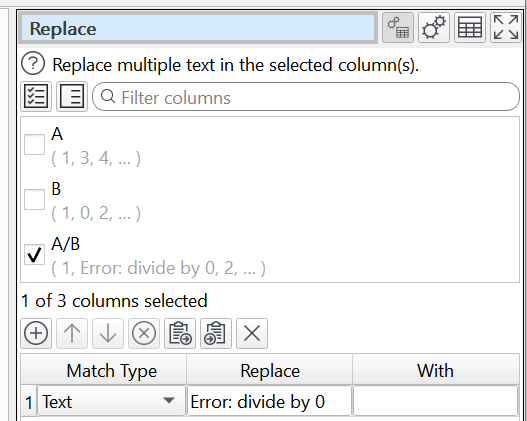# Conditional calculate?

I am trying to get a calculation but only for some rows (category). The problem is that in calculate you divide columns and for some categories, an error is posted (divided by zero by example). I had tried to use a filter to apply only to some categories, but I can’t restore all the values (were filtered).
In my mind, the logic will work with an IF flow transform (that is not implemented as I know). Another option is to use JavaScript, and ask in the JavaScript transform for the category (if the IF could accept calculations…)

Any suggestion?
Regards,
Miguel

You can Filter and then Join back to the original text using a unique key, such as generate by Row Num. But it is a bit of a hassle.

A quick and dirty way to divide column A by column B and not have any warnings is to use Calculate for all rows then Replace to remove any warnings.Or you can do it using the Javascript transform:

dirty-divide.transform (2.6 KB)

JavaScript transform worked smoothly, thanks very much for the input!

Regards,

Miguel

1 Like

Also a quick fix, apply “Num Format”, and set “Non-numeric” to “Change to 0” or “Change to empty”

1 Like

Thanks, it worked very well!

Regards,

Miguel